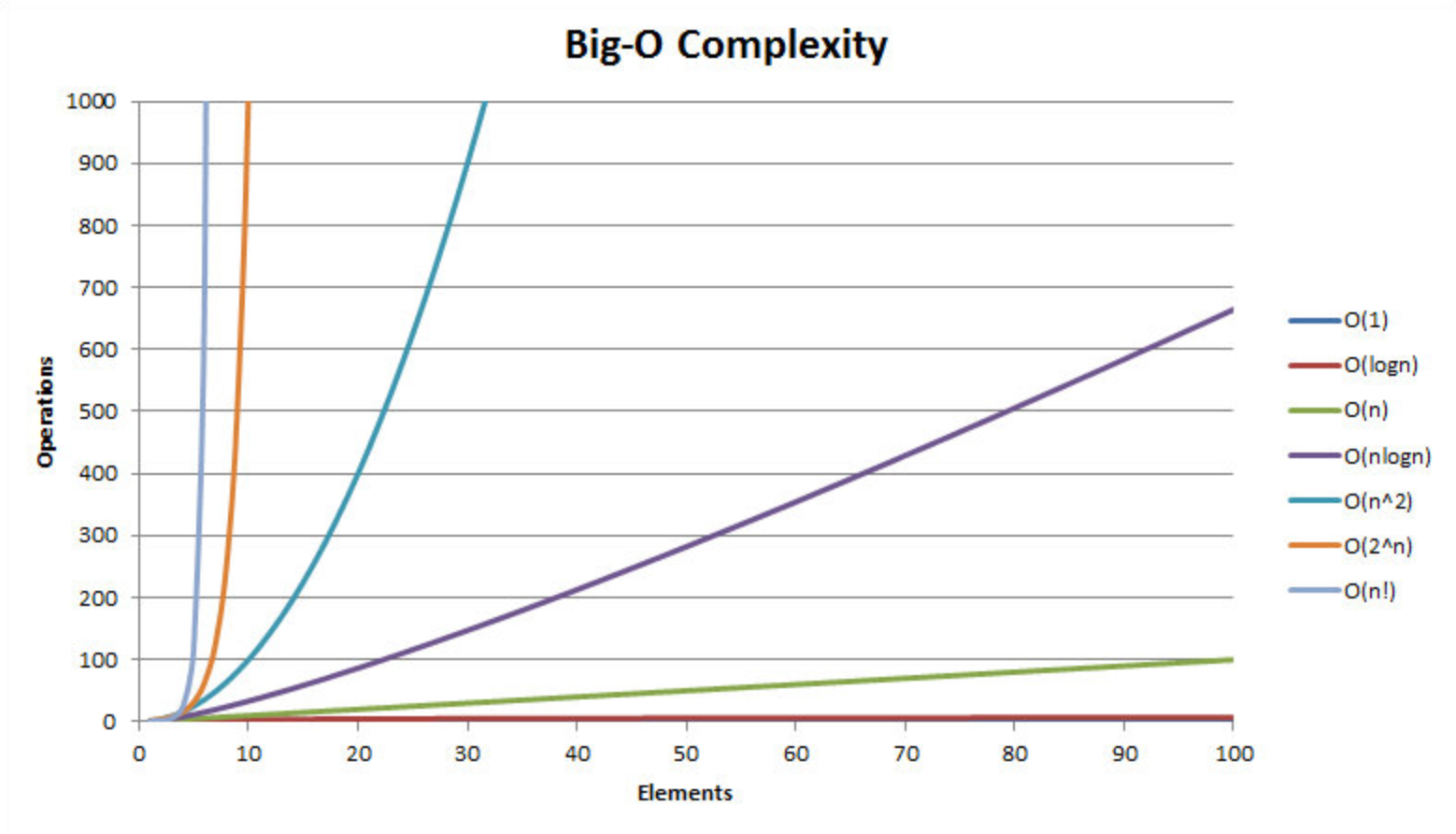星期五, 十二月 25日 2020, 8:00 早上

6.1k 字     22 分钟

# 「算法与数据结构」时间与空间复杂度

## 什么是算法

• 分析问题，选择数据结构，得出初步的解决方法

• 将解决方法合理地拆分，整理成许多步骤

• 为重复的步骤选择合理的循环变量

• 使用易转化为程序实现的自然语言简练地描述算法

• 时间维度：指执行代码所消耗的时间，即时间复杂度
• 空间维度：指执行代码所消耗的空间，即空间复杂度

## 时间复杂度

``````function fn1(){
console.log("句末")
console.log("isboyjc")
}``````

``````function fn2(n){
for(let i = 0; i < n; i++){
console.log("句末")
}
}``````

``````let i = 0
i < n
i++
console.log("句末")``````

`let i = 0` 此条声明语句只在第一次 `for` 循环声明时执行 1 次

`i < n` 此条语句执行次数根据形参 n 的大小变化，`n = 3` 时，即 `i=0,i=1,i=2,i=3` 时会执行，即此条语句执行次数为 `n + 1`

`i++` 此条语句执行次数也是根据形参 n 的大小变化，`n = 3` 时，即 `i=0,i=1,i=2` 时会执行，即 n 次

`console.log("句末")` 此条语句执行次数还是根据形参 n 的大小变化，`n = 3` 会执行 3 次，那么此语句在函数内部即会执行 n 次

``1 + (n + 1) + n + n = (3n + 2)``

``````T(n) = 2            // fn1
T(n) = 3n + 2 // fn2``````

``````// fn1
T(n) = 2

// fn2
T(n) = 3n + 2``````

PS：晓得可能有人把数学知识还给老师了，所以解释下

``T(n) = 10n^4 + 100n^2 + 1000``

`T(n)` 为常数时，时间复杂度为 `O(1)` ，反之时间复杂度为 `O(保留T(n)的最高次项并去掉最高次项的系数)`

``````function fn01(){
console.log("你看这是啥")
console.log("这是一个输出")
console.log("哈哈哈")
let a = 1
return a
}``````

``````function fn02(n){
for(let i = 0; i < n; i++){
console.log("这是一个输出🎓")
}
}``````

``````function fn03(n){
for(let i = 0; i < n; i++){
console.log("外层循环")
for(let j = 0; j < n; j++){
console.log("内层循环")
}
}
}``````

``````function fn04(n){
for(let i = 0; i < n; i++){
console.log("外层循环")
for(let j = 0; j < n; j++){
console.log("内层循环")
}
}

for(let i = 0; i < n; i++){
console.log("哈哈哈")
}
}``````

``````function fn05(n){
for(let i = 0; i < n; i++){
console.log("外层循环")
for(let j = i; j < n; j++){
console.log("内层循环")
}
}
}``````

`i = 0` 时，里层循环会执行 n 次

`i = 1` 时，里层循环会执行 n - 1 次

`i = 2` 时，里层循环会执行 n - 2 次

`i = 3` 时，里层循环会执行 n - 3 次

`i = n - 2` 时，里层循环会执行 2 次

`i = n - 1` 时，里层循环会执行 1 次

``T(n) = n + (n - 1) + (n - 2) + ... + 3 + 2 + 1``

``````S(n) = n*S1 + n*(n - 1)*d/2

// 或

S(n) = n*(S1 + Sn)/2``````

``````// n*(S1 + Sn)/2

n*(n + 1)/2 = (n^2 + n)/2 = (1/2)n^2 + (1/2)n``````

``````function fn06(n){
for(let i = 1; i < n; i *= 2){
console.log("isboyjc")
}
}``````

``````n=2        时打印1次 T(n)=1
n=4        时打印2次 T(n)=2
n=8        时打印3次 T(n)=3
n=16     时打印4次 T(n)=4``````

``````n=2        时打印1次 T(n)=1 // 2^1 = 2
n=4        时打印2次 T(n)=2 // 2^2 = 4
n=8        时打印3次 T(n)=3 // 2^3 = 8
n=16     时打印4次 T(n)=4 // 2^4 = 16``````

PS：又来补数学了

``````function fn07(n,m){
for(let i = 0; i < n; i++){
while(j < m){
console.log("你看懂了吗")
j = j * 2
}
}
}``````• 常数级 `O(1)`
• 对数级 `O(log n)`
• 线性级 `O(n)`
• 线性对数级 `O(n*log n)`
• 平方级 `O(n^2)`
• 立方级 `O(n^3)`
• K次方级 `O(n^k)`
• 指数级 `O(2^n)`

## 空间复杂度

``````function fn001(n){
for(let i = 0; i < n; i++){
console.log("空间复杂度")
}
}``````

``````function fn002(n){
let arr = []
while(n--){
arr.push(n)
}
}``````

## 最后

GitHub算法仓库，从零更算法题/文字/视频 题解ing，来关注吧 👉 GitHub传送门